## How to Count Cells With Text in Excel

Excel spreadsheets are one of the best ways to store and analyze data. Spreadsheets can be made up of cells with a combination of numbers and text. To understand your data more, you may need to differentiate the cells with text.In this article, we’ll show you how to select a cell range then use the COUNTIF function to count the cells with text. In addition, you can learn how to find specific, duplicate, and colored text in your spreadsheet.

## How to Count Cells With Text in Excel on a Windows PC

1. Click on an empty cell on your spreadsheet to insert the formula.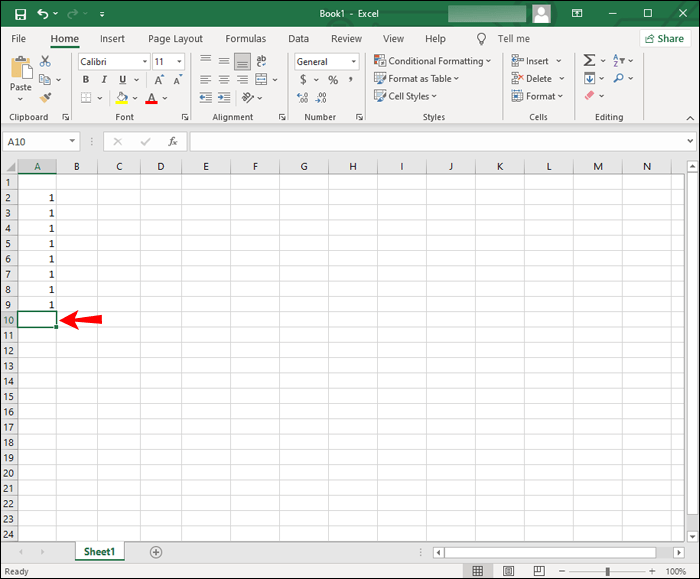2. Type in the empty cell “`=COUNTIF (range, criteria)`”. This formula is for counting the number of cells with text in them within a cell range.3. In “range” enter the cell range you want to check. Enter the first and last cells separated by a colon. For example, to count cells A2 to A9 you’d enter “`A2:A9`”.4. In “criteria” enter “*”. This counts the number of cells with text in the range. The complete formula should look similar to “`=COUNTIF (A2:A9, “*”)`”.5. Now press the enter or return key to apply the formula. The result will display in the formula cell.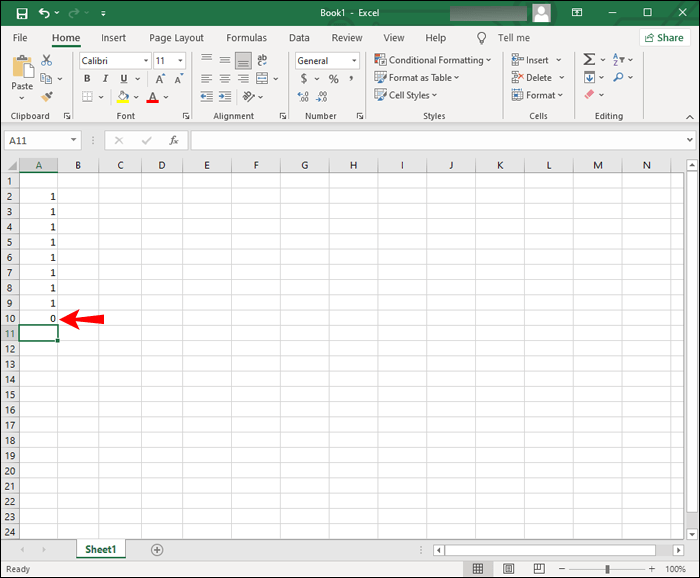## How to Count Cells With Text in Excel on a Mac

The steps for counting the cells with text in your spreadsheet via macOS are the same as with Excel for Windows:

1. Launch Excel then open the spreadsheet you want to analyze.

2. Select an empty cell on your spreadsheet to enter the formula.

3. In the empty cell type “`=COUNTIF (range, criteria)`”. This formula counts the number of cells with text within a cell range.

4. In “range” enter the cell range you want to look at. Enter the first and last cells divided by a colon. For example, to count cells B2 to B10 you’d enter “`B2:B10`”.

5. In “criteria” enter “*”. This counts the number of cells with text in the range. For example, your complete formula should look similar to “`=COUNTIF (B2:B10, “*”)`”.

6. On your keyboard, press the return or enter key to apply the formula. The result will display in the selected cell.

## How to Count Cells With Text in Excel 365

To count the cells with text in your spreadsheet using Excel 365, you’d use the same COUNTIF function used in Excel for Windows and macOS. Here’s how:

1. Open the Excel spreadsheet you wish to examine.

2. Click on an empty cell to type the formula.

3. In the empty cell type: “`=COUNTIF (range, criteria)`”. This formula will count the number of cells with text in them within a specified cell range.

4. In “range” enter the cell range you want to look at. Enter the first and last cells separated by a colon. For example, to count cells C2 to C11 enter “`C2:C1`”.

5. In “criteria” enter “*”. This counts the number of cells with text in the range. For example, your complete formula should look something like “`=COUNTIF (C2:C11, “*”)`”.

6. Now hit the enter or return key to apply the formula. The result will display in the formula cell.

## How to Count Cells With Text in Excel on the iPhone App

To count the number of cells in your spreadsheet with text in them using the Excel app via iPhone:

1. Open the spreadsheet you wish to work on by tapping on “Open” to view your saved spreadsheets, then tap on the file to open it.

2. Double-tap on an empty cell in the spreadsheet to enter the COUNTIF formula. Or you can long-press an empty cell then tap “Edit” from the pop-up menu.3. In the empty cell type, “`=COUNTIF (range, criteria)`”. This formula will count the number of cells containing text inside a cell range.4. For the “range” type the cell range you want to count. Enter the first and last cells separated by a colon. To count cells D2 to D12 enter “`D2:D12`”.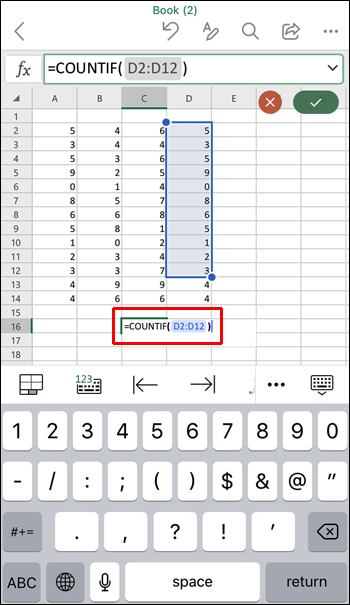5. For the “criteria” type “*”. This counts the number of cells with text in the range. The complete formula should look something like: “`=COUNTIF (D2:D12, “*”)`”.6. Now tap enter or return key to apply the formula. The result will display in the formula cell.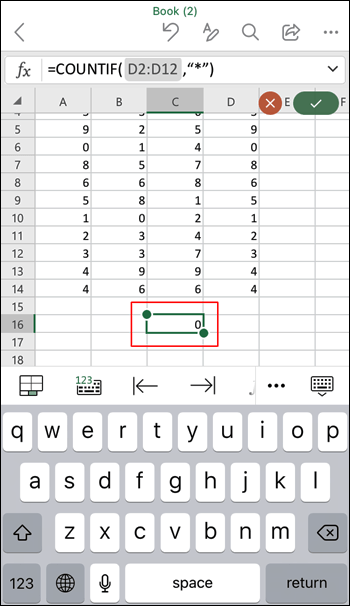## How to Count Cells With Text in Excel on the Android App

To count the number of cells with text in your spreadsheet using the Excel app via your Android device:

1. Open the spreadsheet you want to examine by tapping on “Open” to see your saved spreadsheets, then tap on the file to open it.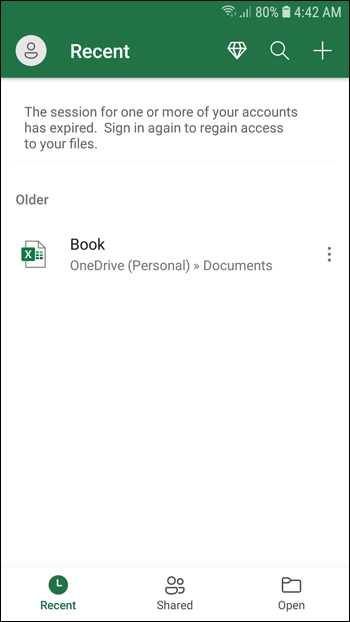2. Double-tap on an empty cell to enter the COUNTIF formula. Alternatively, long-press an empty cell then tap “Edit” from the pop-up menu.3. In the empty cell enter “`=COUNTIF (range, criteria)`”. This formula counts the number of cells with text inside a cell range.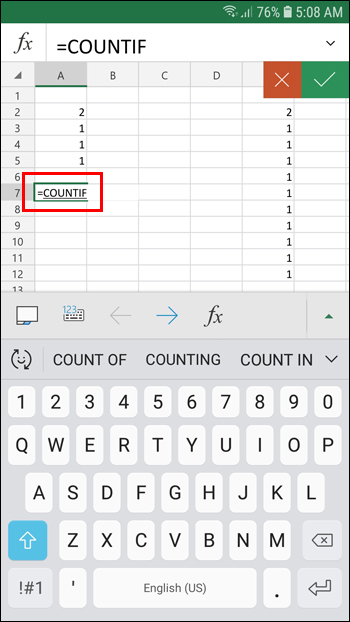4. In “range” enter the cell range you wish to count. Enter the first and last cells separated by a colon. To count cells E2 to E12 enter “`E2: E12`”.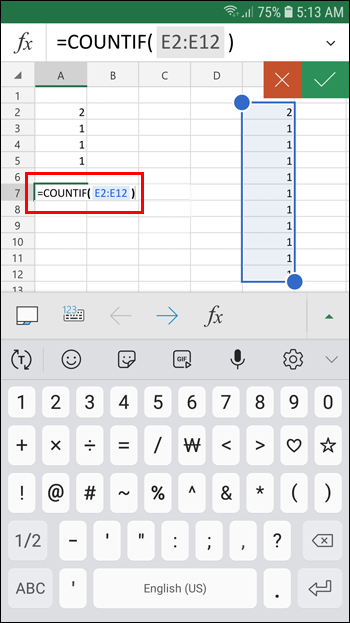5. For the “criteria” type “*”. This counts the number of cells with text in the specified range. Your complete formula should look something like: “`=COUNTIF (A2:E12, “*”)`”.6. Now tap enter or return key to apply the formula. The result will display in the formula cell.## How to Count Cells With Specific Text in Excel

By using the “COUNTIF” function we can count the number of cells with specific text. In this example, we’ll look for the number of times the word “Excel” appears in a specific cell range:

1. Open the spreadsheet in Excel you wish to examine.

2. Click on an empty cell to type the formula.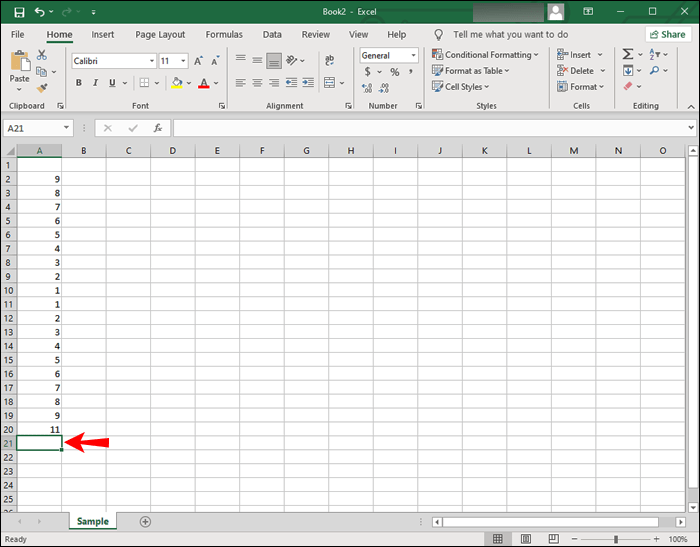3. In the empty cell type “`=COUNTIF (range, criteria)`”. This formula will count the number of cells containing specific text within a cell range.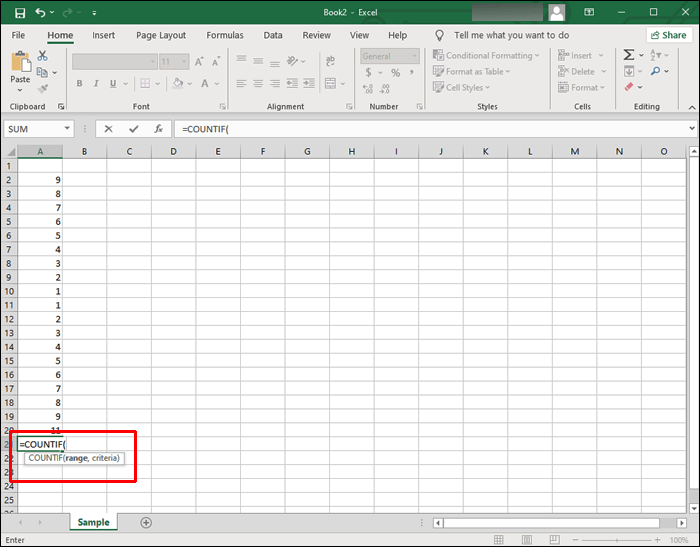4. For the “range” enter the cell range you wish to count. Enter the first and last cells separated by a colon. To count cells A2 to A20 enter “`A2: A20`”.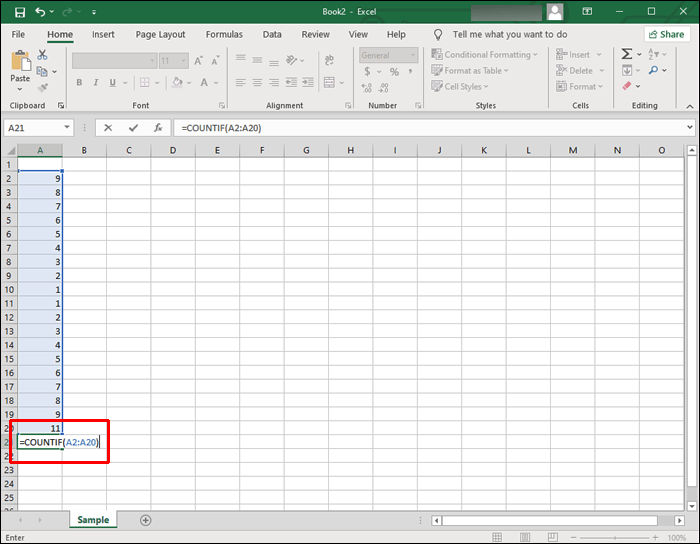5. For the “criteria” type “Excel.” This counts the number of cells with “Excel” in the specified range. Your formula should look something similar to “`=COUNTIF (A2:A20, “Excel”)`”.## How to Count Cells With Duplicate Text in Excel

In the following example, we’re looking for duplicate student grades. Our spreadsheet is set up as follows:

• Column A – lists our students A2:A10

• Column B – lists each student’s grades (A, B, or C)

• Column D –lists the available grades. D2 for “As,” D3 for “Bs,” and D4 for “Cs.”

• Column E – lists the count of each grade.

### Count Cells With Duplicate Text Including First Instance

To count the number of cells in your spreadsheet with instances of grade “A,” “B,” or “C” – including the first instance – enter the following formulas:

• For instances of grade “A,” click cell E2 and type formula “`=COUNTIF (B2 : B10, D2)`”.• For instances of grade “B,” click cell E3 and type formula “`=COUNTIF (B2 : B10, D3)`”.• For instances of grade “C,” click cell E4 and type formula “`=COUNTIF (B2 : B10, D4)`”.Now you have the count for duplicate grades including the first instance listed in column “E.”

### Count Cells With Duplicate Text Excluding First Instance

To count the number of cells in your spreadsheet with instances of grade “A,” “B,” or “C” – excluding the first instance – enter the following formulas:

• For instances of grade “A” excluding the first instance, click cell E2 and type formula “`=COUNTIF (\$B\$2 : \$B\$10, D2)-1`”.• For instances of grade “B” excluding the first instance, click cell E3 and type formula “`=COUNTIF (\$B\$2 : \$B\$10, D3)-1”.`• For instances of grade “C” excluding the first instance, click cell E4 and type formula “`=COUNTIF (\$B\$2 : \$B\$10, D4)-1`”.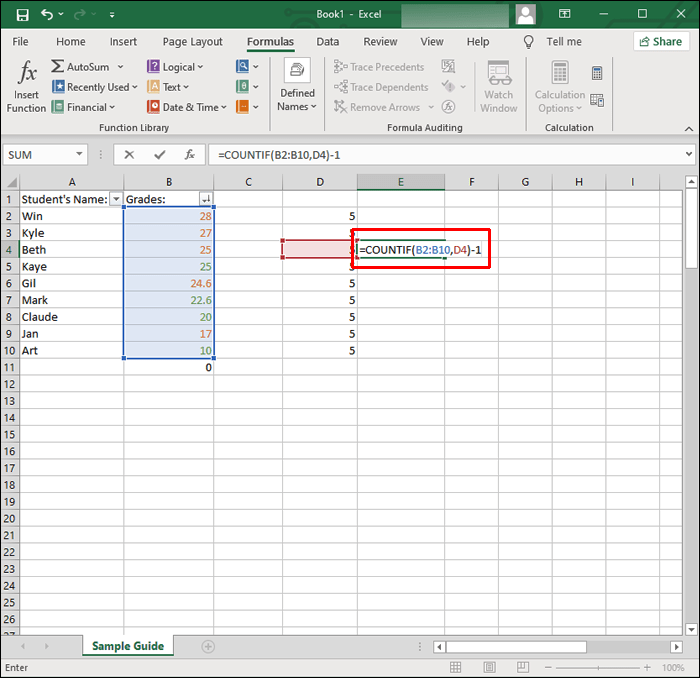Now you have the count for duplicate grades excluding the first instance listed in column “E.”

## How to Count Cells With Color Text in Excel

Excel does not have a formula to count cells based on their text color. To get around this, filter the results then count. Here’s how:

1. Open the spreadsheet you wish to analyze.2. Right-click a cell with the text of the color you wish to count.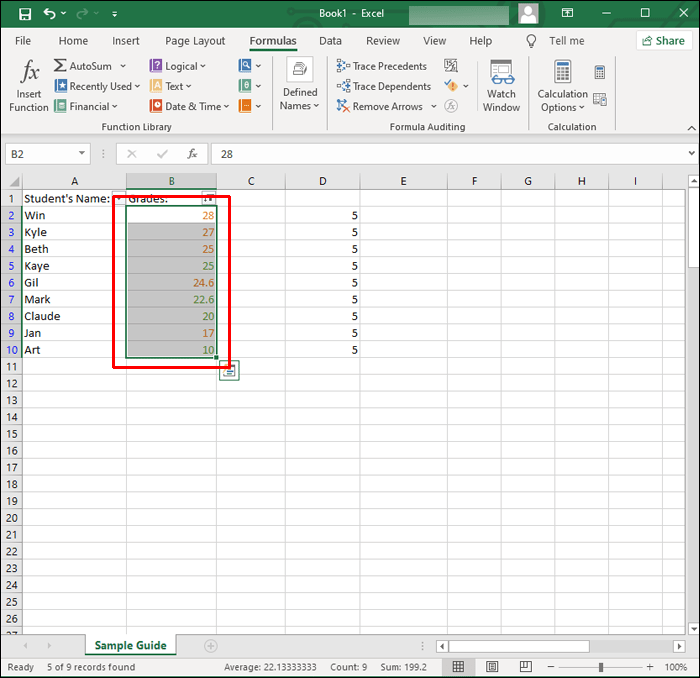3. Select “Filter,” then “Filter by Selected Cell’s Font Color” to filter the cells with the selected text color.4. Next, tell Excel to count your data range. If your text is listed from cell B2 to B20 enter the following formula: “`=SUBTOTAL (B2 : B20)`”.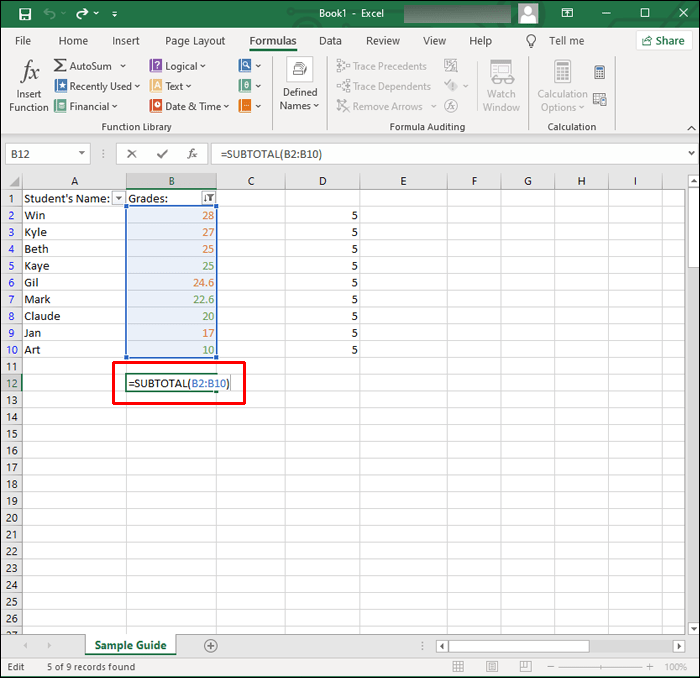Once you hit “Enter” to apply the filter, Excel will only display the cells containing that color and hide the remaining values.

The “SUBTOTAL” function will exclude the values in the hidden rows, therefore, returning the count for the selected text color only.

## Finding the Cells With Text

The Excel application does a great job of storing your data and facilitating analysis. It handles text as well as numbers.

Its over four hundred functions include the “COUNTIF” function. This function is useful for finding the total of cells containing specific information like cells with text, and the number of occurrences for specific text.

Were you able to find out what you needed to know about your spreadsheet data? How useful do you find Excel in general? Share your opinions in the comments section below.